##### Managerial Economics For Dummies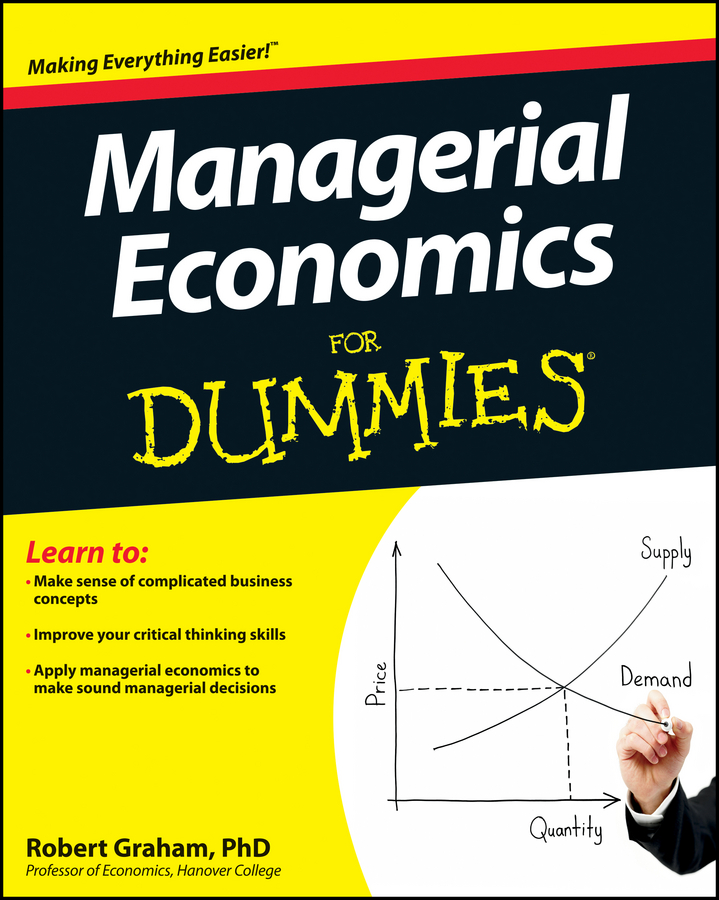Unfortunately, it isn’t always the case that the firm’s profit is positive. Nevertheless, firms may continue producing in the short run in order to minimize losses. It’s important to remember that firms who shut down in the short run still have production costs — total fixed cost can’t be changed.

Thus, if a firm loses less money than total fixed cost by producing in the short run, the firm should continue production in order to minimize losses.

The illustration shows a situation where a firm minimizes losses by producing in the short run. The profit-maximizing quantity of output is still determined by equating marginal revenue and marginal cost. The firm produces the profit-maximizing quantity of output q0 at that point.

However, because price is less than average total cost at q0, the firm loses money. Its loss per unit equals price minus average total cost. This loss per unit is represented by the double-headed arrow.

If instead of producing q0 the firm shuts down, it loses total fixed cost. Total fixed cost equals average fixed cost multiplied by the quantity of output, and average fixed cost equals average total cost minus average variable cost. Thus, the difference between average total cost, ATC0, and average variable cost, AVC0, represents the fixed cost per unit at q0.

This difference between average total cost and average variable cost is clearly larger than the difference between price and average total cost. Thus, shutting down and losing your fixed costs is a greater loss than occurs if you produce q0.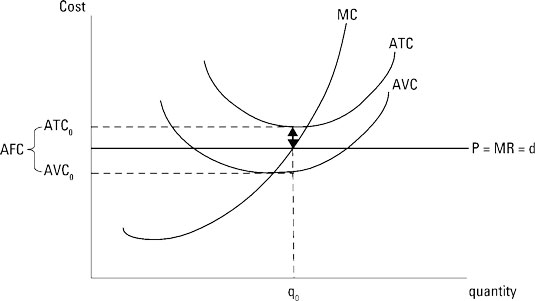Assume the market-determined price for your good is \$6.80. Therefore, your total revenue equals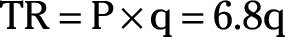Marginal revenue equals the derivative of total revenue taken with respect to quantity or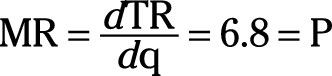If your total cost function is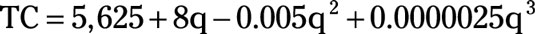Marginal cost equals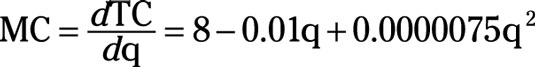In order to determine the profit-maximizing quantity of output, you simply set marginal revenue or price equal to marginal cost and solve for q.

1. Set marginal revenue equal to marginal cost.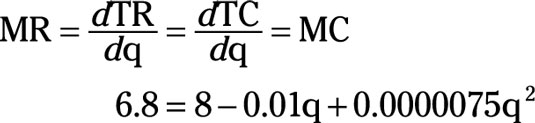2. Solve for q.

Use either a calculator or the quadratic formula.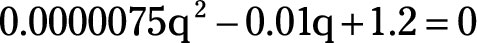The profit maximizing quantity of output is 1,200 units.

3. Determine the average total cost equation.

Average total cost equals total cost divided by the quantity of output.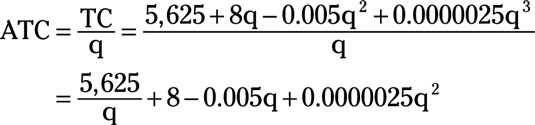4. Substitute the profit-maximizing quantity of 1,200 for q to determine average total cost.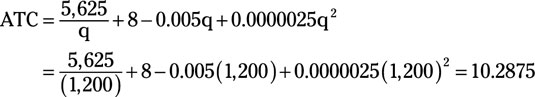5. Calculate profit per unit.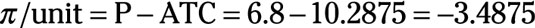or profit per unit equals –\$3.4875.

6. Determine total profit by multiplying profit per unit by the profit-maximizing quantity of output.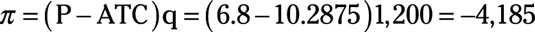or total profit equals –\$4,185. Your firm is losing \$4,185. But note that if you immediately shut down, your losses equal total fixed cost, which is \$5,625. Losing \$4,185 is a bad situation, but losing \$5,625 is even worse. Continuing production makes the best of a bad situation.